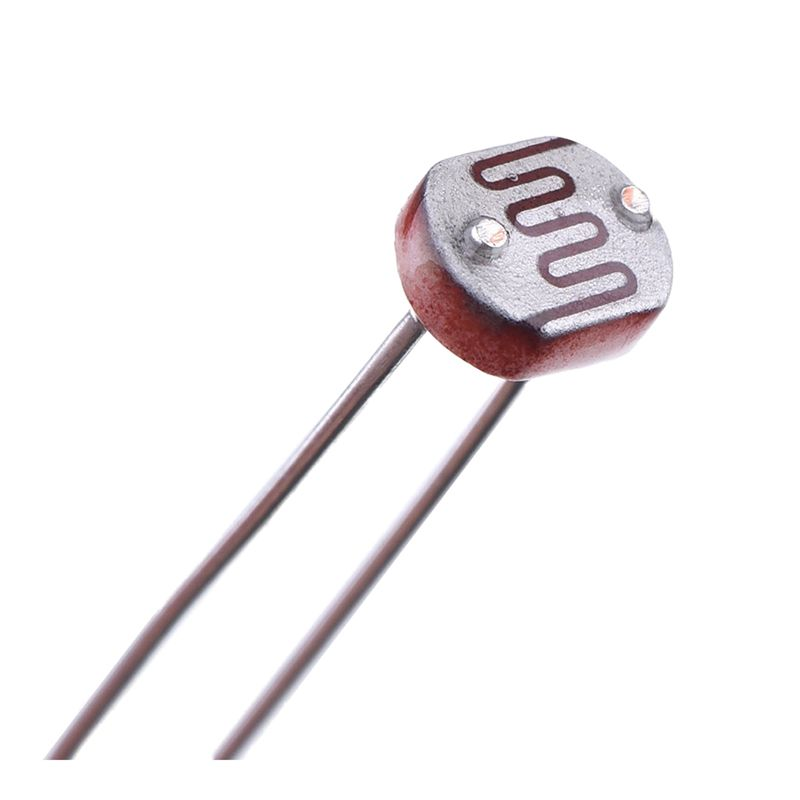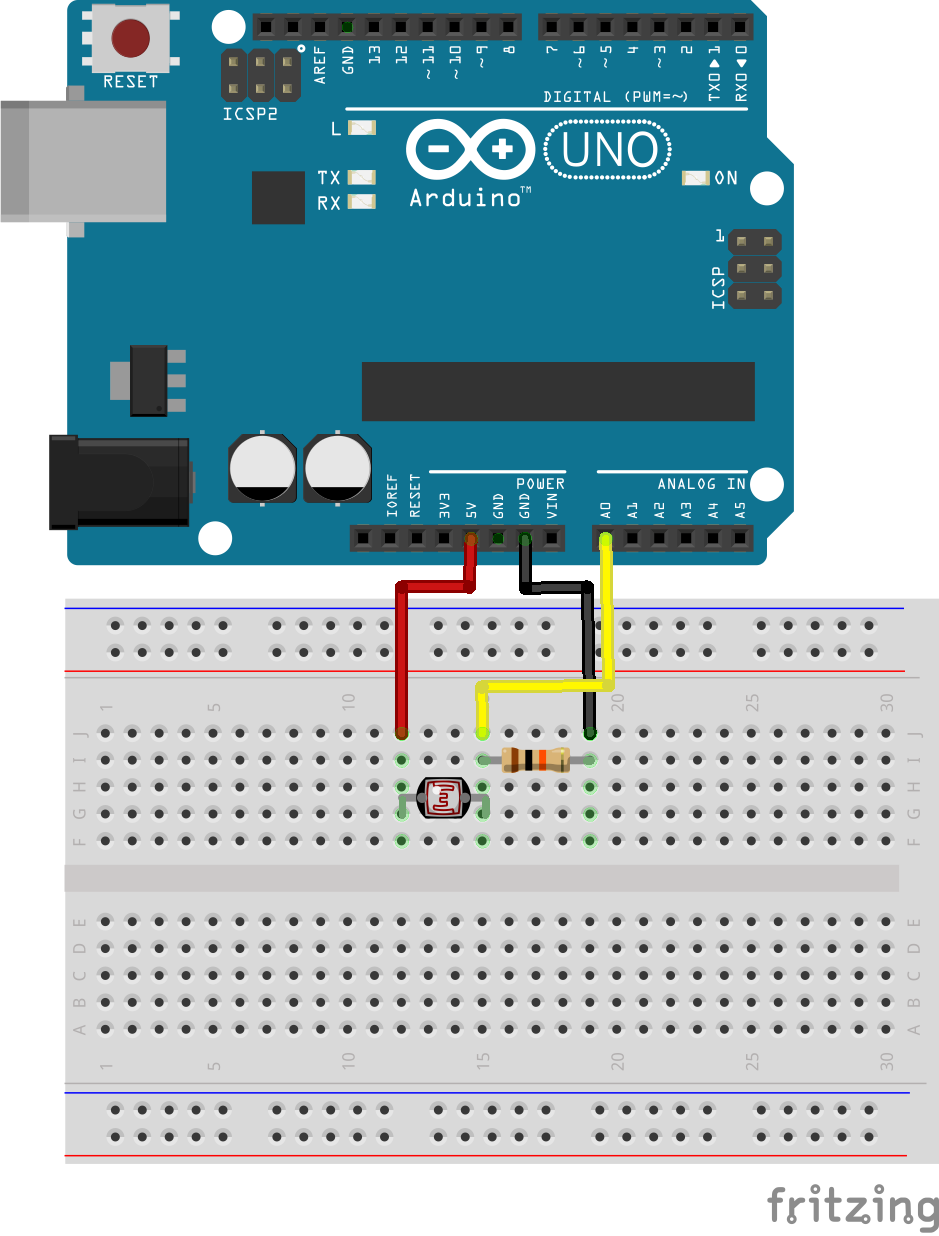# What is an LDR?

An LDR or Light Dependent Resistor is a component which restricts how much power can flow through a circuit based on how much or little light hits the sensitive part on the top.To use a Light Dependent Resistor, we have to use it in combination with a fixed value resistor, the combination of these two components acts a little like a kitchen mixer tap, we can vary the temperature (voltage) by adjusting the tap.The zig-zag lines indicate resistors, the voltage output we measure with the Arduino comes from this middle point between the two, as the value of the LDR varies it changes the voltage between Ground (0V) and 5V (VCC).

# Wiring

1. (1)leg to 5V (Power)
2. (2)leg split to GND via 10K resistor
3. (2)leg split to A0# Getting started

The following code uses `analogRead()` to get a integer between 0-1023 representing the voltage, where 0 is 0V and 1023 is 5V.

The code below uses the serial port to output the value every 50ms to the Serial Monitor.

``````#define ldrPin A0

void setup() {
Serial.begin( 9600 );
pinMode( ldrPin, INPUT );
}

void loop() {